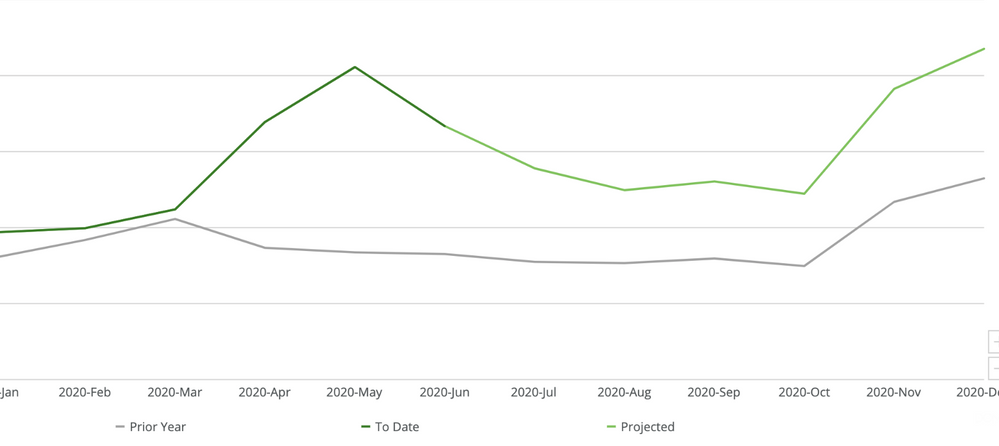# Different Date Label on a single chart

I am trying to show a monthly/quarterly trend chart showing actual and forecast numbers. Is there anyway to label the date for actual and forecast differently? For the year of 2020, Jan through June would be labeled a regular date, but July 2020 and forward will be labeled something like July-2020(F) and so on.

Thanks

Juan

• How I've done graphs like that in the past was to have two different lines plotted on the same graph and color code them differently while labeling one Actual and the other Forecast.

To get around some odd graphing issues I had the actual stop at the current day (I was graphing on a by day granularity - you can adjust however granular you want - concept would remain the same). For the forecast values I actually ended up setting the Actual values up to the current day and then had projected values for today going forward in time. This would cause the data to draw directly on top of each other so there was a single line and since the actual values stopped it then only showed the projected values in the future.I also ended up displaying numbers that were projected with an asterisk using some beast modes (special comma formatting because Domo doesn't have to_char SQL function):

```CONCAT(CASE WHEN LENGTH(ROUND(SUM(`Number Orders`), 0)) = 12 THEN
CONCAT(
SUBSTRING(ROUND(SUM(`Number Orders`), 0), 1, 3), ',',
SUBSTRING(ROUND(SUM(`Number Orders`), 0), 4, 3), ',',
SUBSTRING(ROUND(SUM(`Number Orders`), 0), 7, 3), ',',
SUBSTRING(ROUND(SUM(`Number Orders`), 0), 10, 3)
)
WHEN LENGTH(ROUND(SUM(`Number Orders`), 0)) = 11 THEN
CONCAT(
SUBSTRING(ROUND(SUM(`Number Orders`), 0), 1, 2), ',',
SUBSTRING(ROUND(SUM(`Number Orders`), 0), 3, 3), ',',
SUBSTRING(ROUND(SUM(`Number Orders`), 0), 6, 3), ',',
SUBSTRING(ROUND(SUM(`Number Orders`), 0), 9, 3)
)
WHEN LENGTH(ROUND(SUM(`Number Orders`), 0)) = 10 THEN
CONCAT(
SUBSTRING(ROUND(SUM(`Number Orders`), 0), 1, 1), ',',
SUBSTRING(ROUND(SUM(`Number Orders`), 0), 2, 3), ',',
SUBSTRING(ROUND(SUM(`Number Orders`), 0), 5, 3), ',',
SUBSTRING(ROUND(SUM(`Number Orders`), 0), 8, 3)
)
WHEN LENGTH(ROUND(SUM(`Number Orders`), 0)) = 9 THEN
CONCAT(
SUBSTRING(ROUND(SUM(`Number Orders`), 0), 1, 3), ',',
SUBSTRING(ROUND(SUM(`Number Orders`), 0), 4, 3), ',',
SUBSTRING(ROUND(SUM(`Number Orders`), 0), 7, 3)
)
WHEN LENGTH(ROUND(SUM(`Number Orders`), 0)) = 8 THEN
CONCAT(
SUBSTRING(ROUND(SUM(`Number Orders`), 0), 1, 2), ',',
SUBSTRING(ROUND(SUM(`Number Orders`), 0), 3, 3), ',',
SUBSTRING(ROUND(SUM(`Number Orders`), 0), 6, 3)
)
WHEN LENGTH(ROUND(SUM(`Number Orders`), 0)) = 7 THEN
CONCAT(
SUBSTRING(ROUND(SUM(`Number Orders`), 0), 1, 1), ',',
SUBSTRING(ROUND(SUM(`Number Orders`), 0), 2, 3), ',',
SUBSTRING(ROUND(SUM(`Number Orders`), 0), 5, 3)
)
WHEN LENGTH(ROUND(SUM(`Number Orders`), 0)) = 6 THEN
CONCAT(
SUBSTRING(ROUND(SUM(`Number Orders`), 0), 1, 3), ',',
SUBSTRING(ROUND(SUM(`Number Orders`), 0), 4, 3)
)
WHEN LENGTH(ROUND(SUM(`Number Orders`), 0)) = 5 THEN
CONCAT(
SUBSTRING(ROUND(SUM(`Number Orders`), 0), 1, 2), ',',
SUBSTRING(ROUND(SUM(`Number Orders`), 0), 3, 3)
)
WHEN LENGTH(ROUND(SUM(`Number Orders`), 0)) = 4 THEN
CONCAT(
SUBSTRING(ROUND(SUM(`Number Orders`), 0), 1, 1), ',',
SUBSTRING(ROUND(SUM(`Number Orders`), 0), 2, 3)
)
WHEN LENGTH(ROUND(SUM(`Number Orders`), 0)) <= 3 THEN
ROUND(SUM(`Number Orders`), 0)
WHEN SUM(`Number Orders`) IS NULL THEN 0
ELSE ''
END
, CASE WHEN MAX(`Data Type`) = 'Projected' THEN '*' ELSE '' END)```

You might be able to do something similar with the dates where you just concatenate your date with a case statement. Also - sorting may get wonky so you might need to sort by the date field in your chart as well.

My Data was under the format of:

Date | Value | Type

1/1/2020 | 456 | Actual

1/1/2020 | 789 | Actual

**Did this solve your problem? Accept it as a solution!**
• @GrantSmith  would it make sense to stack the data

Such that you have

ACTUAL

UNION

FORECAST

UNION

LAST YEAR OFFSET

with a column 'Activity Type' to differentiate the three sets of data.  Then use a CASE statement to differentiate between SUM(CASE WHEN Date <= today() then Actual ..) and SUM(CASE WHEN Date > today() then Forecast)

That way you don't have to differentiate the data in ETL and you can compare the forecast from last week against the actual from last week when you get around to evaluating the accuracy of your forcast.

Jae Wilson
Check out my 🎥 Domo Training YouTube Channel 👨‍💻

**Say "Thanks" by clicking the ❤️ in the post that helped you.
**Please mark the post that solves your problem by clicking on "Accept as Solution"
• @jaeW_at_Onyx - That's how my data is formatted, it's utilizing the Type column to determine if it's either a projection or an actual number. I'm not tracking historical projections (it's forward looking only) so I'm not comparing old projections (It's simply just multiplying the YoY % over the last X weeks and then multiplying last years data by that percentage - very simplistic model).

The projections are calculated in the ETL but everything else is done at the card level (using the case statements like you mentioned)